# Arithmetic - math word problems

#### Number of problems found: 2634

• 3y inflation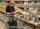Price of the roll rise in the first year by 9%, the second year fell by 5% and in the third year increased by 3%. Calculate the average annual increase in price of the roll.
• Big factorial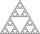How many zeros end number 116! ?
• InflationOnce upon a time, tsar owned a money printer and printed and printed. The result of printing money prices went up,in the first year 3.9 %, in the second 6%, in the third 4.7% and in the fourth 5.5%. Then tsar was failed in election. Calculate the average
• Triangle ABCRight triangle ABC with right angle at the C, |BC|=18, |AB|=33. Calculate the height of the triangle hAB to the side AB.
• Insurance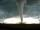The house owner is insured against natural disasters and pays 0.04% annually of the value of house 53 Eur. Calculate the value of the house. Calculate the probability of disaster if you know that 41% of the insurance is to pay damages.
• MonkeyThe monkey fell in 40 meters deep well. Every day it climbs 3 meters, at night it dropped back by 2 m. On what day it gets out from the well?
• Cone in cylinder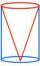The cylinder is inscribed cone. Determine the ratio of the volume of cone and cylinder. The ratio express as a decimal number and as percentage.
• Logarithmic equation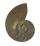Solve equation: log13(7x + 12) = 0
• Number ratio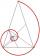Calculate two positive numbers that its ratio is 6:6 and difference was 0.
• ShapePlane shape has a maximum area 677 mm2. Calculate its perimeter if perimeter is the smallest possible.
• Pension or fraud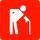Imagine that your entire working life working honestly and pay taxes and to Social Insurance (in Slovakia). You have a gross wage 900 Euros, you pay social insurance (you and your employer) monthly € 288 during 41 years and your retirement is 405 Eur
• Farmer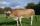The farmer farming 293 ha of arable land and the remaining 20% are meadows and pastures. How much land farmer owns?
• Drunk man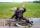Drunkman has 2.6‰ of alcohol in the blood. How many grams of alcohol has in blood, if he have 5 kg of blood?
• Rhombus problem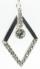The perimeter of the rhombus is 268 m, and its area is 2278 m2. Calculate the height of the rhombus.
• Cube wallThe surface of the first cube wall is 64 m2. The second cube area is 40% of the surface of the first cube. Determine the length of the edge of the second cube (x).
• Circle chordWhat is the length x of the chord circle of diameter 115 m if the distance from the center circle is 11 m?
• Triangle SASCalculate the triangle area and perimeter, if the two sides are 51 cm and 110 cm long and angle them clamped is 130 °.
• Square diagonalCalculate the length of the square diagonal if the perimeter is 172 cm.
• Rectangle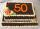Area of rectangle is 3002. Its length is 41 larger than the width. What are the dimensions of the rectangle?
• Chickens and rabbitsIn the yard were chickens and rabbits. Together they had 18 heads and 56 legs. How many chickens and how many rabbits were in the yard?

Do you have an exciting math question or word problem that you can't solve? Ask a question or post a math problem, and we can try to solve it.

We will send a solution to your e-mail address. Solved examples are also published here. Please enter the e-mail correctly and check whether you don't have a full mailbox.